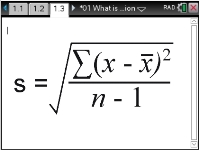Activities

•• Subject Area

• Standard: VCE: Further Mathematics: Further Mathematics

• AuthorAust Senior

30 Minutes

• Device
• TI-Nspire™ CAS
• TI-Nspire™ Navigator™
• Software

TI-Nspire™ CAS
TI-Nspire™ CAS Navigator™ NC System

4.2

What is Standard DeviationActivity Overview

Students investigate the idea of ‘standard deviation – what does it mean, and how is affected by the distribution of a statistical variable.

Objectives

• Introduction of the idea of the variation in the values of a numerical statistical variable
• What is standard deviation
• How does the distribution of the variable affect the value of the standard deviation

Vocabulary

statistical variable, mean, standard deviation